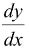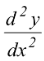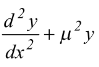# Formation of differential equation

## Formation of differential equation

The differential equation of a family of curves of one parameter is a differential equation of the first order, the differential equation of a family of curves of two parameters is a differential equation of the order two and the differential equation of a family of curves of n parameters is a differential equation of n order.

If family of curves have one parameter then we differentiate it once and eliminate parameter using equation of family of curves and equation we get after differentiation. For example x2 + y2 = a2 (a is a parameter), represents family of concentric circles.

x2 + y2 = a2 (1)

Differentiate (1)

2x + 2y.= 0 (2)

⇒ xdx + ydy = 0 is differential equation.

Family of curves of two parameters will be differentiated twice to get a relation independent of any parameter.

Similarly for family of curves of n parameters will be differentiated n times and then eliminate all the parameters.

For example

y = a sin μx + b cos μx (a, b are parameters)= aμcosμx − bμsinμx= −aμ2sinμx − bμ2cosμx= −μ2 (a sinμx + b cosμx)= 0 is differential equation.